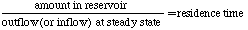## Residence Time

This is a good opportunity to discuss the notion of a residence time for a reservoir. The residence time is effectively the average length of time that an entity, in this case a water molecule, will remain in a reservoir. It is really only meaningful for a reservoir that is at or near a steady state condition. By definition, the residence time is the amount of material in the reservoir, divided by either the inflow or the outflow (they are equal when the reservoir is at equilibrium). If there are multiple inflows or outflows, then we use the sum of the outflows or inflows to determine the residence time. For our bathtub system here, the residence time is thus 10 liters divided by 1 liter per second, which is equal to 10 seconds. It is fairly easy to see that if we increase the flow rates, the water moves through the reservoir faster, so the residence time decreases. In the form of an equation, this definition is expressed as:It is possible then, to determine any of the above three parameters if the other two are known and if we assume the system is in a steady state. For instance, if we assume that the human population is in a steady state (of course it is not), and if we know the average lifespan, we can calculate the number of births and deaths in a year. The population is 5.6 billion, so if we assume an average lifespan of 70 years, then we can say that 80 million people are born each year and 80 million people die each year, assuming a steady state.